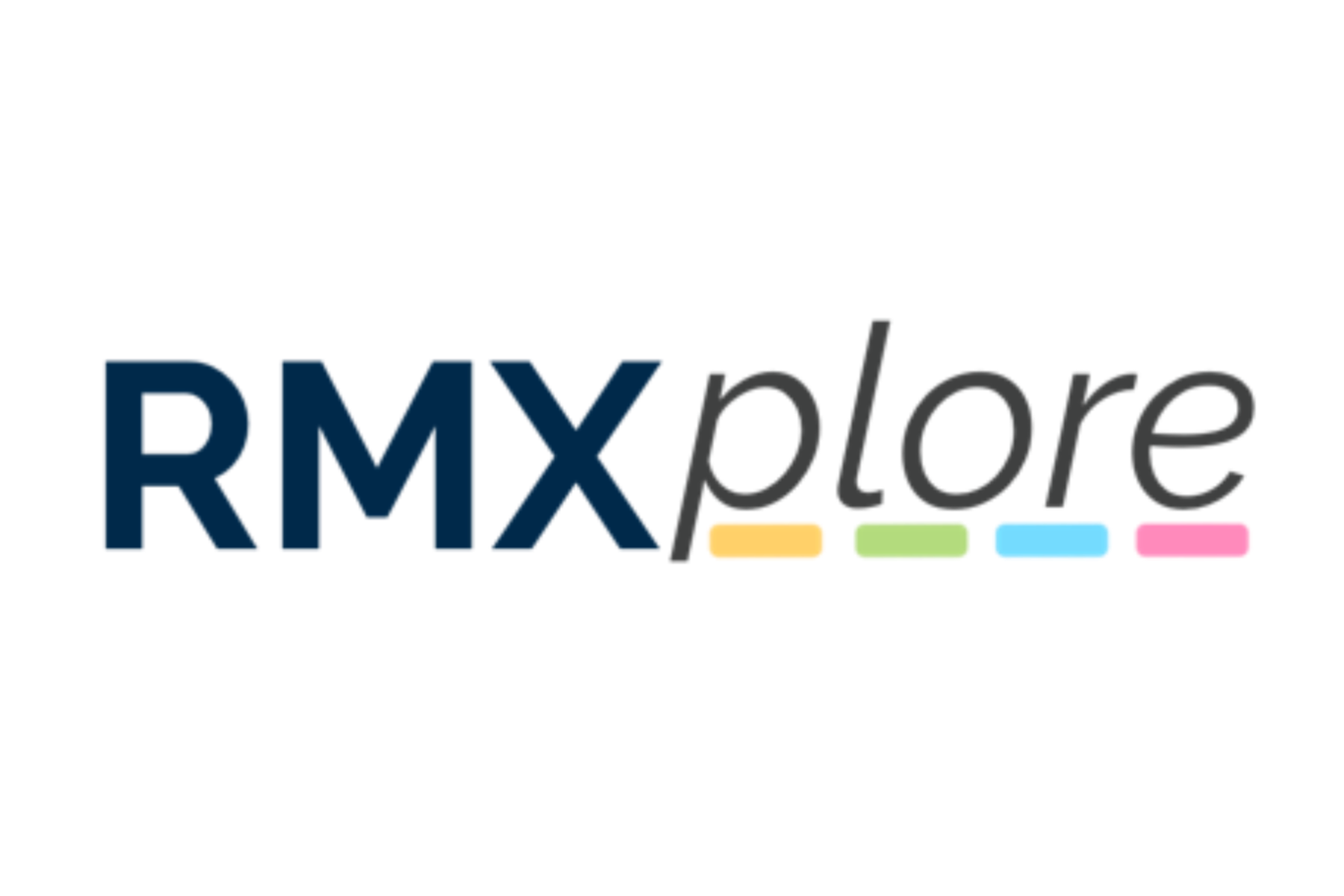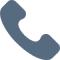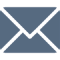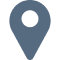# Algebra II

Please note that RMXplore's Math Bytes Courses are not
currently open for registration
.

### Algebra II

Coming Soon!
Course Pricing:
• Online : \$260
• Houston Center : \$320
• Cupertino Center : \$400
Course Logistics:
Duration : to be updated
Number of Classes: 5 weeks course
Occurs : to be updated

### Math Byte Course Topics:

Course Topics are organized as six modules with five granular topics covered under each module. Click through below to see all modules/topics for this course.

#### 01 - Relations and Functions• Polynomial Division
• Remainder Theorem
• Rational Root Theorem
• Fundamental Theorem of Algebra
• Graphing Rational and Polynomial Functions

#### 02 - Polynomial and Rational Functions• Polynomial Division
• Remainder Theorem
• Rational Root Theorem
• Fundamental Theorem of Algebra
• Graphing Rational and Polynomial Functions

#### 03 - Sequences and Series• Expressing series in sigma notation
• Arithmetic Sequences
• Geometric Sequences
• Arithmetico-Geometric Series
• Sums of Common Series

#### 04 - Complex Numbers• Standard form for a complex number
• Conjugates and its applications
• Magnitude and argument
• The complex plane
• Polar form of complex numbers

#### 05 - Conic Sections• Identifying Conic Types
• Parabola: foci, directrices and the eccentricity
• Ellipse: foci, directrices and the eccentricity
• Hyperbola: foci, directrices and the eccentricity
• Circles

#### 06 - Probability and Statistics• Sets and Venn Diagrams
• Permutations
• Combinations
• Introduction to Probability
• Binomial Theorem